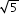# MA.8.GR.1.2

Apply the Pythagorean Theorem to solve mathematical and real-world problems involving the distance between two points in a coordinate plane.

### Examples

The distance between (-2,7) and (0,6) can be found by creating a right triangle with the vertex of the right angle at the point (-2,6). This gives a height of the right triangle as 1 unit and a base of 2 units. Then using the Pythagorean Theorem the distance can be determined from the equation 1²+2²=c², which is equivalent to 5=c². So, the distance isunits.

### Clarifications

Clarification 1: Instruction includes making connections between distance on the coordinate plane and right triangles.

Clarification 2: Within this benchmark, the expectation is to memorize the Pythagorean Theorem. It is not the expectation to use the distance formula.

Clarification 3: Radicands are limited to whole numbers up to 225.

General Information
Subject Area: Mathematics (B.E.S.T.)
Strand: Geometric Reasoning
Date Adopted or Revised: 08/20
Status: State Board Approved

## Related Courses

This benchmark is part of these courses.
1205050: M/J Accelerated Mathematics Grade 7 (Specifically in versions: 2014 - 2015, 2015 - 2020, 2020 - 2022, 2022 and beyond (current))
1205070: M/J Grade 8 Pre-Algebra (Specifically in versions: 2014 - 2015, 2015 - 2022, 2022 and beyond (current))
1204000: M/J Foundational Skills in Mathematics 6-8 (Specifically in versions: 2014 - 2015, 2015 - 2022, 2022 and beyond (current))
7812030: Access M/J Grade 8 Pre-Algebra (Specifically in versions: 2014 - 2015, 2015 - 2018, 2018 - 2019, 2019 - 2022, 2022 and beyond (current))

## Related Access Points

Alternate version of this benchmark for students with significant cognitive disabilities.
MA.8.GR.1.AP.2: Given the Pythagorean Theorem, determine lengths/distances between two points in a coordinate system by forming right triangles, with natural number side lengths.

## Related Resources

Vetted resources educators can use to teach the concepts and skills in this benchmark.

## Formative Assessments

Distance Between Two Points:

Students are asked to find the distance between two points in the coordinate plane.

Type: Formative Assessment

Distance on the Coordinate Plane:

Students are asked to find the distance between two points in the coordinate plane.

Type: Formative Assessment

Coordinate Plane Triangle:

Students are asked to determine the lengths of the sides of a right triangle in the coordinate plane given the coordinates of its vertices.

Type: Formative Assessment

Calculate Triangle Sides:

Students are asked to determine the length of each side of a right triangle in the coordinate plane given the coordinates of its vertices.

Type: Formative Assessment

## Lesson Plan

Just Plane Ol' Area!:

Students will construct various figures on coordinate planes and calculate the perimeter and area. Use of the Pythagorean theorem will be required.

Type: Lesson Plan

## Perspectives Video: Professional/Enthusiast

What's the Distance from Here to the Middle of Nowhere?:

Find out how math and technology can help you (try to) get away from civilization.

Download the CPALMS Perspectives video student note taking guide.

Type: Perspectives Video: Professional/Enthusiast

## Text Resource

Pythagoras Explained:

This informational text resource is intended to support reading in the content area. The text describes a method for predicting the win-loss record for baseball teams based on runs scored and runs allowed, using the "Pythagorean Expectation" formula invented by Bill James. The text goes on to show the relationship of the prediction formula to the Pythagorean theorem, pointing out a very cool application of the theorem to the world of sports.

Type: Text Resource

## MFAS Formative Assessments

Calculate Triangle Sides:

Students are asked to determine the length of each side of a right triangle in the coordinate plane given the coordinates of its vertices.

Coordinate Plane Triangle:

Students are asked to determine the lengths of the sides of a right triangle in the coordinate plane given the coordinates of its vertices.

Distance Between Two Points:

Students are asked to find the distance between two points in the coordinate plane.

Distance on the Coordinate Plane:

Students are asked to find the distance between two points in the coordinate plane.

## Student Resources

Vetted resources students can use to learn the concepts and skills in this benchmark.

## Perspectives Video: Professional/Enthusiast

What's the Distance from Here to the Middle of Nowhere?:

Find out how math and technology can help you (try to) get away from civilization.

Download the CPALMS Perspectives video student note taking guide.

Type: Perspectives Video: Professional/Enthusiast

## Parent Resources

Vetted resources caregivers can use to help students learn the concepts and skills in this benchmark.

## Perspectives Video: Professional/Enthusiast

What's the Distance from Here to the Middle of Nowhere?:

Find out how math and technology can help you (try to) get away from civilization.

Download the CPALMS Perspectives video student note taking guide.

Type: Perspectives Video: Professional/Enthusiast